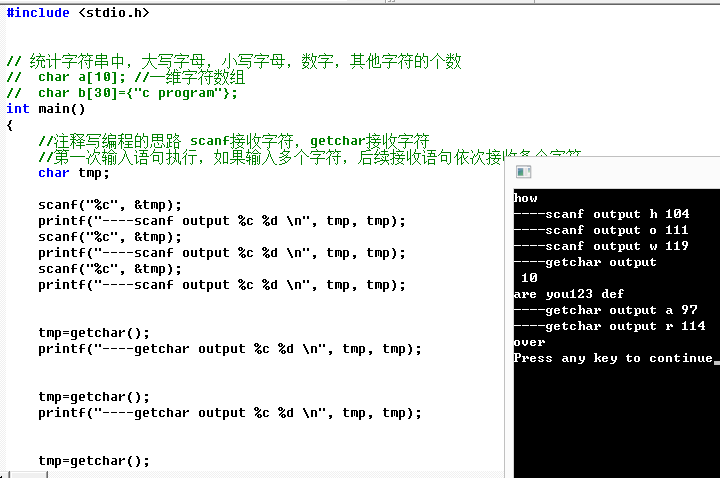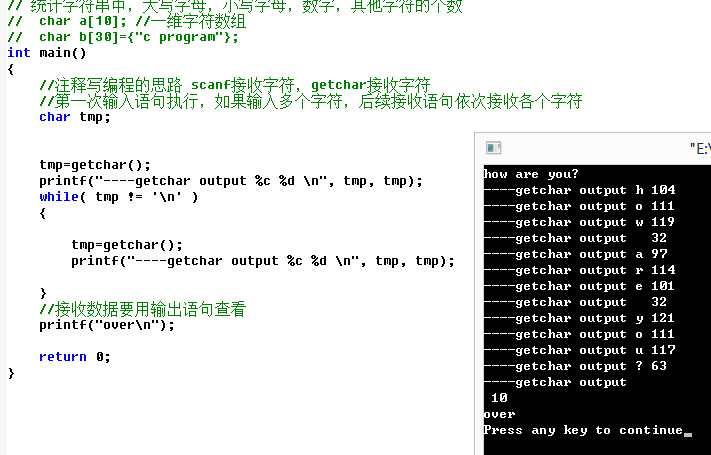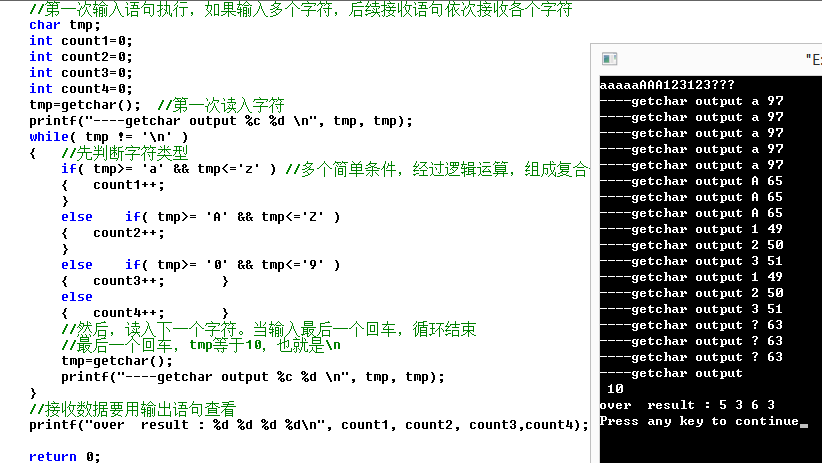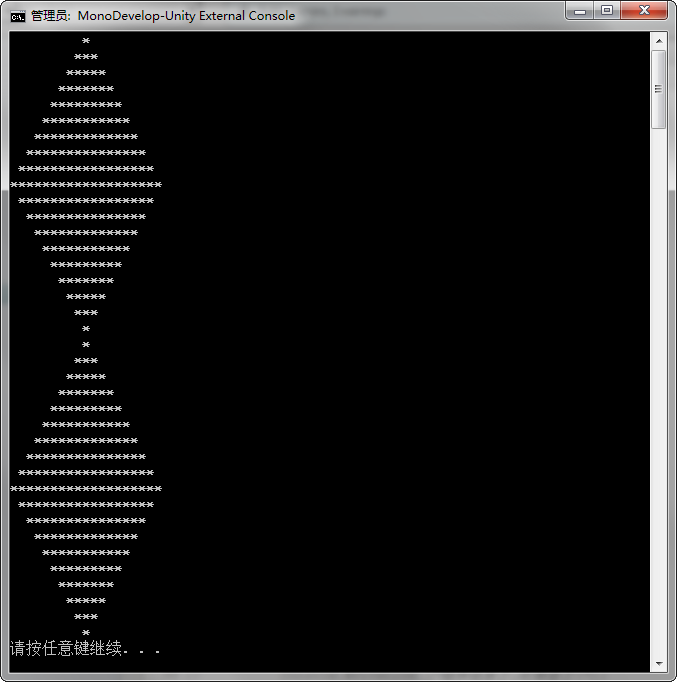• 多加输出语句查看变量。 1. 连续的输入语句scanf,getchar, 会把中途的空格、回车键都读入变量。 2. getchar+while，连续读入多个字符，直到按回车键。 3.多分支判断字符类型。 具体截图如下，后面有视频讲解地址...
编程习惯：在注释写编程思路。多加输出语句查看变量。
1. 连续的输入语句scanf,getchar, 会把中途的空格、回车键都读入变量。
2. getchar+while，连续读入多个字符，直到按回车键。
3.多分支判断字符类型。
具体截图如下，后面有视频讲解地址。
我的主要工作是讲java,jsp，但是，C语言是基本功，不能丢。视频下载地址：
链接: https://pan.baidu.com/s/1Om-ctqVlAtmS8YiSW0F5kw 提取码: yw5y 
展开全文c语言 getchar scanf
• ## php循环语句输出

千次阅读 2018-11-12 10:07:52
//输出html 结构 <label for="a1">IT1 foreach ($out as$key => $value) { # code... echo "<li>"; echo "$value['c_id']."' type='checkbox' name='chk_art' value='...
1.使用$mysqli <?php require '../data/conn.php';$sql = 'SELECT * FROM zy_category';
$resulet =$mysqli->query($sql);$out = [];
while (true) {
$row =$resulet->fetch_assoc();
if ($row == null) break;$out[] = $row; } ?> //。。html //输出html 结构 <ul class="checkbox-container" id="checkboxContainer"> <li> <input id="a1" type="checkbox" name="chk_art" value="it1"> <label for="a1">IT1</label> </li> <?php foreach ($out as $key =>$value) {
# code...
echo "<li>";
echo "<input id='a".$value['c_id']."' type='checkbox' name='chk_art' value='".$value['article_type']."'>";
echo "<label for='a".$value['c_id']."'>".$value['article_type']."</label>";
echo "</li>";
}
for ($i=0;$i < count($out);$i++) {
# code...
// echo "for..".$out[$i]['article_type'];
}
?>

</ul>

展开全文php
• shell编程循环语句与函数一 echo语句1.1 echo常用转义字符1.2 运算方法二 循环语句2.1 for循环2.1.1 for循环格式2.1.2 循环中断（break、continue）2.1.3 for循环三种方法2.2 while循环2.3 until循环三 函数3.1 函数...


shell编程循环语句与函数
一 echo语句1.1 echo常用转义字符1.2 运算方法
二 循环语句2.1 for循环2.1.1 for循环格式2.1.2 循环中断（break、continue）2.1.3 for循环三种方法
2.2 while循环2.3 until循环
三 函数3.1 函数返回值3.2 递归函数3.3 函数库的创建与调用
四 for循环输出九九乘法表、菱形4.1 乘法表4.2 菱形

一 echo语句
1.1 echo常用转义字符
echo -n表示不换行输出 echo -e输出转义字符，将转义后的内容输出到屏幕上
常用转义字符释义\b转义后相当于按退格键(backspace) ，但前提是"\b"后面存在字符; “\b"表示删除前一个字符，”\b\b"表示删除前两个字符。\c不换行输出，在"\c"后面不存在字符的情况下，作用相当于echo -n; 但是当"\c"后面仍然存在字符时，"\c"后面的字符将不会被输出。\n换行，被输出的字符从"\n"处开始另起一行。\v或\f换行，但是换行后的新行的开头位置连接着上一行的行尾\t表示插入tab，即横向制表符;\r光标移至行首，但不换行，相当于使用"\r"以后的字符覆盖"\r"之前同等长度的字符,但是当"\r"后面不存在任何字符时，"\r" 前面的字符不会被覆盖\\表示插入“\”本身
操作实例1.2 运算方法
shell脚本中有4种运算方法
1. i=$(expr 12 \* 5) 2. i=$((12*5))
3. i=$[12*5] 4. let i= 12*5 i++相当于i=$[$i+1] i--相当于i=$[$i-1] i+=2相当于i=$[$i+2]  二 循环语句 2.1 for循环 使用for循环语句时，需要指定一个变量及可能的取值列表，针对每一个不同的取值重复执行相同的命令序列，直到变量值用完完全退出循环。在这里，取值列表是for语句的执行条件，其中包括多个属性相同的对象，需要预先指定。 2.1.1 for循环格式for 变量名 in 取值列表 do 命令序列 done  例：添加用户和删除用户 #!/bin/bash添加用户 ULIST=$(cat /root/user.txt)
for UNAME in $ULIST do useradd$UNAME
echo "123456" | passwd --stdin $UNAME &> /dev/null //把输出信息丢弃 done  #!/bin/bash删除用户$ULIST=$(cat /root/user.txt) for UNAME in$ULIST
do
userdel -r $UNAME [$? -eq 0] && [echo "已删除用户$UNAME"] done  2.1.2 循环中断（break、continue） break默认跳出单个循环，break 2跳出两个循环，以此类推，break n跳出n个循环。 continue终止某次循环中的命令，但不会完全终止整个循环 2.1.3 for循环三种方法 方法1 [root@localhost ~]#for i in {1..10};do echo$i;done		//按序输出1到10
[root@localhost ~]#for i in {1..10..2};do echo $i;done //输出1到10，步长为2 方法2 [root@localhost ~]#for i in$(seq 1 10);do echo $i;done //按序输出1到10 [root@localhost ~]#for i in$(seq 1  2 10);do echo $i;done //输出1到10，步长为2 方法3 [root@localhost ~]#for ((i=1;i<=10;i++));do echo$i;done

2.2 while循环while 条件测试操作
do
命令序列
done

例：竞猜商品价格
PRICE=$(expr$RANDOM % 1000)
TIMES=0
echo "good's price between 0-999,how much?"
while true
do
let TIMES++
if [ $INT -eq$PRICE ];then
echo "You're right.The price is $PRICE" echo "You have guessed$TIMES times."
exit 0
elif [ $INT -gt$PRICE ];then
echo "Too high!"
else echo "Too low!"
fi
done

2.3 until循环
重复测试某个条件，只要条件不成立就反复执行until 条件测试操作
do
命令序列
done

例：从1开始累加
#!/bin/bash
i=0;s=0
until [ $i -eq$num ]
do
let "i++";let "s=$s+$i"
done
echo "sum(1...$num)="$s

三 函数
函数定义
[function] 函数名 ()
{
命令序列
[return x]
}

调用函数
函数名 [参数1] [参数2]

3.1 函数返回值
return表示退出函数并返回一个退出值，脚本中可以用

?

变

量

显

示

该

值

使

用

原

则

：

1

函

数

一

结

束

就

取

返

回

值

，

因

为

?变量显示该值 使用原则： 1 函数一结束就取返回值，因为

?变量只返回执行的最后一条命令的退出状态码 2 退出状态码必须是0-255，超出时将除以256取余
db()
{
return $[$value * 2]
}
db
echo $?  3.2 递归函数 递归函数调用自己本身，在分析函数时可以先跳过函数调用，再对该部分进行分析。 例：递归遍历环境变量中的目录和可执行文件 #!/bin/bash //递归函数部分 file_list() { for f in$1/*		// $1/*表示取值列表，读取PATH下目录 do if [ -d$f ];then		//对$f进行判断，-d表示是否为目录 echo "$2$f" file_list "$f" " $2" //调用函数自身，把$f下的所有目录和文件作为取值列表，再次进行判断

elif [ -x $f ];then //对$f进行判断，-x表示是否为可执行文件
echo "$2$f"		//输出空格接该文件
fi
done
}
//主程序部分
IFS=$IFS':' //IFS字段分隔符，默认包含空格，换行，制表符，加入':'对冒号也可以识别并进行分隔 for folder in$PATH
do
echo "$folder:" file_list "$folder" " "		//调用函数，加入位参1，2
done

3.3 函数库的创建与调用
例：加减乘除函数库
创建函数库
[root@localhost ~]#vim myfuncs.sh
jiafa()
{
echo $[$1+$2] } jianfa() { echo$[$1-$2]
}

chengfa()
{
echo $[$1*$2] } chufa() { if [$2 -ne 0 ];then
echo $[$1/$2] else echo '$2不能为0'
fi
}

//调用函数库
. myfuncs.sh

value1=10
value2=5
result1=jiafa $value1$value2
result2=jianfa $value1$value2
result3=chengfa $value1$value2
result4=chufa $value1$value2
echo "加法结果为$result1" echo "减法结果为$result2"
echo "乘法结果为$result3" echo "除法结果为$result4"

四 for循环输出九九乘法表、菱形
4.1 乘法表
#!/bin/bash
for ((i=1;i<=9;i++))		//外循环控制行数
do
for ((j=1;j<=i;j++))		//内循环控制列数
do
echo -n -e "$i x$j = $((i * j))\t" done echo -e "\n" done4.2 菱形 #!/bin/bash #菱形上半部分 for ((i=9;i>=1;i--)) #控制行数 do for ((a=1;a<=$i;a++))         #控制第i行第一段输出
do
echo -n " "
done
for ((b=9;b>=$i;b--)) #控制第i行第二段输出 do echo -n "*" done for ((c=8;c>=$i;c--))         #控制第i行第三段输出
do
echo -n "*"
done
echo ""
done

#菱形下半部分
for ((h=2;h<=9;h++))
do
for ((d=1;d<=$h;d++)) do echo -n " " done for ((e=9;e>=$h;e--))
do
echo -n "*"
done

for ((f=8;f>=$h;f--)) do echo -n "*" done echo "" done展开全文linux shell bash • 学习第二天了,今天写一下当时小难了我一下的代码,用循环语句输出一个由*组成的菱形;输出结果:自己忙活了一个小时,最后发现老师的方法比我简便多了,今天就把两种方法都写出来吧!其实是类似的方法第一种方法:public ...  学习第二天了,今天写一下当时小难了我一下的代码,用循环语句输出一个由*组成的菱形; 输出结果:自己忙活了一个小时,最后发现老师的方法比我简便多了,今天就把两种方法都写出来吧!其实是类似的方法 第一种方法: public void lingxing (){ //第一种方法 for (int a = 1; a <= 10; a++) { for (int b = 9; b >= a; b--) { Console.Write (" "); } for (int c = 1; c <= a; c++) { Console.Write ("*"); } for (int e = 1; e<a ; e++) { Console.Write ("*"); } for (int f = 9; f >= 1; f--) { Console.Write (" "); } Console.WriteLine (); } for (int a = 1; a <= 9; a++) { for (int b = 1; b <= a; b++) { Console.Write (" "); } for (int c = 9; c >= a; c--) { Console.Write ("*"); } for (int b = 8; b >= a; b--) { Console.Write ("*"); } for (int c = 1; c <= a; c++) { Console.Write (" "); } Console.WriteLine (); } 自己写的,代码多,有点杂乱. 这个采用的方法是把这个菱形分成四块儿来实现了,比较麻烦,看着也有点乱 第二种方法: 这是老师给的答案: for (int a = 1;a <=10;a++){ for (int b = 1; b <=(10 - a); b++) { Console.Write (" "); } for (int c = 1; c <= 2 * a - 1; c++) { Console.Write ("*"); } Console.WriteLine (); } for (int a = 9;a >=1;a--){ for (int b = 1; b <=(10-a); b++) { Console.Write (" "); } for (int c = 1; c <=2*a -1; c++) { Console.Write ("*"); } Console.WriteLine (); }  展开全文c# • Shell编程循环语句与函数一、echo语句1.1 echo常用转义字符1.2 运算方法二 、循环语句2.1 for循环2.1.1 for循环格式2.1.2 循环中断（break、continue）2.1.3 for循环三种方法2.2 while循环2.3 until循环三 、函数3.1...shell • 这篇文章主要介绍了Python编程中的for循环语句学习教程,是Python入门学习中的基础知识,需要的朋友可以参考下 Python for循环可以遍历任何序列的项目，如一个列表或者一个字符串。 语法： for循环的语法格式如下： ...python •$n=5; //选择打印层数 for($i=1;$i for($k=1;$k  //打印空格 echo " "; } for($j=1;$j  //打印 * 和内部 空格 if($i==1||$i==$n){ echo "*"; }else{ if($j==1||$j==2*$i-1){ echo '*'; }else{ ...}
• C程序设计：用C语言循环语句输出九九乘法表 要求：利用for循环输出九九乘法表； 工具：VC++ 6.0 代码如下： //打印九九乘法表； #include <stdio.h> int main() { int i,j; for(i=1; i<=9; i++){ for...c语言 程序设计 经验分享 程序人生 深度学习
• 代码如下： public class TriangTest { public static void main(String[] args) { int n = 4;//最大三角形的行数 for(int i = 0; i ; i++) { for(int x = i + 1; x ; x+
• 题目：在屏幕上输出形如以下图形，第一行的起如字母和终止字母由用户输入。 A B C D E B C D E A C D E A B D E A B C E A B C D /*第一种实现方法--使用数组*/voidmain(void){charch;inti,j;charch1;/*读入第...
• 循环语句  循环语句在程序设计中用来描述有规则重复的流程。在实际的程序中，存在很多需要重复执行的流程，为了简化这些重复的执行流程，在程序设计语言中新增了该类语句。  在学习循环语句时，最重要的就是发现...
• SAS学习笔记之《SAS编程与数据挖掘商业案例》（4）DATA步循环与控制、常用全程语句输出控制1. 各种循环与控制DO组 创建一个执行语句块 DO循环 根据下标变量重复执行DO和END之间的语句 DO WHILE 重复执行直到条件为...数据挖掘
• Shell编程循环语句（详细讲解以及脚本案例） 文章目录一、for语句的结构（一）、for语句应用实例1、示例1——批量添加用户2、示例2——使用根据IP地址检查主机状态3、示例3——for循环计算1-100奇数之和二、while...shell linux
• ## Python循环语句

千次阅读 2015-08-20 18:29:58
循环语句允许我们执行一个语句或语句组多次，下图是大多数编程语言中的循环语句的一般形式： Python中只提供了for循环和while循环，没有do…while循环。循环控制语句 循环控制语句可以更改语句执行的顺序，...python break
• 特点：先判断条件，条件为真，执行循环语句。条件为假，直接结束循环语句。 语法： while(条件表达式) {  循环语句;//如果条件为真，执行循环语句，如果条件为假，直接结束整个while语句 } while循环执行流程：...while循环 for循环
• ## Python 循环语句

千次阅读 2016-09-10 11:27:40
概述Python提供了for循环和while循环（在Python中没有do..while循环）while循环语法Python 编程中 while 语句用于循环执行程序，即在某条件下，循环执行某段程序，以处理需要重复处理的相同任务。其基本形式为：...
• while循环语句也称前测试循环语句，它的循环重复执行次数，是利用一个条件来控制是否继续重复执行这个语句。while语句与for循环语句相比，无论是语法还是执行的流程，都比较简明易懂。while循环格式如下：  while ...
• ## for循环语句

千次阅读 2013-11-06 07:44:05java
• 循环语句 循环语句在程序设计中用来描述有规则重复的流程。在实际的程序中，存在很多需要重复执行的流程，为了简化这些重复的执行流程，在程序设计语言中新增了该类语句。 在学习循环语句时，最重要的就是发现流程...java eclipse 语言 c
• Python学习（5）一、python的while 循环语句二、python的赋值运算符三、python的while 循环语句 示例四、python的 break 和 continue五、python的while 嵌套1、用嵌套打印小星星2、python 九九乘法表六、python的...python
• 1.输出倒正三角形结果如下图所示： ************* *********** ********* ******* ***** *** * 具体代码如下：public class Test2 {public static void main(String[] args) { int line=20;//定义行数 fjava
• /* 需求：打印一个正立的直角三角形。 * ** *** **** ***** 行数确定了， 列数没有确定？ 分析找出规律： i = 0 ; i<5 ; j=0 , j<=0; 一个星号 ... public static void main(String[] .java
• 2:循环语句(掌握) ( 1)有三种:for,while,do...while (2)for循环语句 A:格式 for(初始化语句;判断条件语句;控制条件语句){ 循环体语句; } 执行流程： a:执行初始化语句 b:执行判断条件...程序员
• 实现从终端获取一条语句，计算这条语句中的空格、字母、数字的个数，统计这条语句全部字符的数量，实现多次输入，并统计一共输入的字符的数量。LinuxC语言 函数
• ## 10.循环语句

千次阅读 多人点赞 2020-11-23 23:00:48
循环：事物周而复始的运动或变化。 《明日边缘》 ... 为了让我们的程序重复执行某些指令，我们就需要用到循环语句。...在Python中的循环语句主要...当条件表达式为真时，程序执行循环语句，其执行流.
• 标题 编程利用循环打印如下字符图形： 12345678987654321 234567898765432 3456789876543 45678987654 567898765 6789876 78987 898 9c语言
• ## Java的循环语句

千次阅读 2014-04-21 21:01:21
Java 的循环语句有for，while 和 do-while 。这些语句创造了我们通常所称的循环（loops）。你可能知道，一个循环重复执行同一套指令直到一个结束条件出现。你将看到，Java 有适合任何编程所需要的循环结构。 ...
• 本文是phython基础语法第二章判断语句和循环语句知识点小结，欢迎阅读，共同进步 文章目录一.if判断语句基本格式介绍二.比较(即关系，条件)运算符三.if-else四.if...elif...else...语句格式五.if嵌套六.While循环...python java...